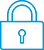cancel
Showing results for
Search instead for
Did you mean:It has been a while since anyone has replied. Simply ask a new question if you would like to start the discussion again.New member
1 0 0 0
Message 1 of 1
347
Flag Post

# Having Trouble getting clean derivatives out of the HP Prime CaclulatorHP Recommended
Hp Prime

Just purchased the HP Prime for my Calc 2 class because the Nspire is banned during testing.  When using the derivative template as so, d e^tan(2x) / dx produces an unsimplified mess for a relatively easy derivative... is this the type of answer that is expected using this calculator?  Also had trouble taking derivatives of x with fraction exponents such as x^3/2, which also produced another headache of an answer.  The equation was entered exactly d 2/3*(x)^3/2 / dx.  The only way I was able to get to the answer needed was taking the answer it originally produced from the equation, simplifing it, approximating it, and then dividing it by x as the calculator did not cancel out the x from top and bottom for some reason.  The answer that should be produced is 1/x^1/3

Tags (1)
† The opinions expressed above are the personal opinions of the authors, not of HP. By using this site, you accept the Terms of Use and Rules of Participation